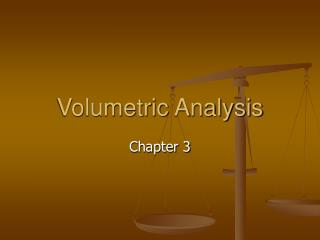DownloadDownload PresentationVolumetric Analysis

Volumetric Analysis

Télécharger la présentationVolumetric Analysis

- - - - - - - - - - - - - - - - - - - - - - - - - - - E N D - - - - - - - - - - - - - - - - - - - - - - - - - - -
Presentation Transcript

1. Volumetric Analysis Chapter 3

2. Concentration • What equation do we know involving concentration? • n = cV • Concentration is expressed in mol L-1, which is given the symbol M • Another measure for concentration is mass per unit volume, g L-1, this is found easily by dividing the mass (in g) by the volume (in L). • To convert mol L-1 to g L-1 multiple the molarity by the molar mass of the solute

3. Your Turn • Page 34 • Question 11a • Question 13 • Question 14b,c

4. Standard Solution • A solution with an accurately known concentration is called a standard solution. • You can not simply make a standard solution by dissolving a measured mass of a substance in water to give a known volume of solution. It just doesn’t always work. • Only a few chemicals encountered can do this, many chemicals are impure as they react with the atmosphere or decompose

5. Standard Solutions • Substances that are so pure that the amount of substance, in mole, can be calculated accurately from their mass are called primary standards. • A primary standard should: • Be readily obtainable in pure form • Have a known formula • Be easy to store without deteriorating or reacting with the atmosphere • Have a high molecular mass to minimise the effect of errors with weighing • Be inexpensive

6. Standard Solutions • Page 29 look at some examples of primary standards.

7. Your Turn • Page 34 • Question 12a

8. Volumetric Analysis • Helps us to find the amount of a substance in solution • Volumetric analysis involves reacting a measured volume of a standard solution with a measured volume of the solution of unknown concentration • Ideally the solutions are mixed until they have just reacted completely in the mole ratio indicated by the stoichiometric equation. • What is this process called????

9. Volumetric Analysis • Turn to page 30 and 31

10. Equivalence point • The equivalence point is the point during the titration when the solutions have been mixed in the mole ratio shown by the reaction equation. • Example 2HCl(aq) + Ca(OH)2(aq) → CaCl2(aq) + H2O(l) The equivalence point is reached when the mole ratio n(HCl) : n(Ca(OH)2) = 2:1 So n(HCl) = 2n(Ca(OH)2)

11. Equivalence point and end point • An indicator must be used in acid-base titrations to detect the equivalence point. • The change in colour during a titration is the end point • So you need an indicator with an end point which closely matches the equivalence point

12. Accuracy • There are always errors associated with measurements made during experimental work. • Typical undertainties associated with volumetric analysis are: • 20 mL pipette ±0.05 mL • Burette ±0.02 mL for each reading • 250.0 mL volumetric flask ±0.3 mL

13. Accuracy in titrations • We always do 3 concordant titres. Why? • This is because the volume of a single drop from a burette is about 0.05 mL • So each concordant titre is within a drop of each other. • Remember we always use the average titre

14. Your Turn • Page 34 • Question 23 For homework Page 34 Question 16 and 21